Next: Sawtooth waves and symmetry Up: Classical waveforms Previous: Classical waveforms   Contents   Index

Symmetries and Fourier series

Before making a quantitative analysis of the Fourier series of the classical waveforms, we pause to make two useful observations about symmetries in waveforms and the corresponding symmetries in the Fourier series. First, a Fourier series might consist only of even or odd-numbered harmonics; this is reflected in symmetries comparing a waveform to its displacement by half a cycle. Second, the Fourier series may contain only real-valued or pure imaginary-valued coefficients (corresponding to the cosine or sine functions). This is reflected in symmetries comparing the waveform to its reversal in time.

In this section we will assume that our waveform has an integer period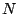, and furthermore, for simplicity, thatis even (if it isn't we can just up-sample by a factor of two). We know from Chapter 9 that any (real or complex valued) waveform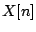can be written as a Fourier series (whose coefficients we'll denote by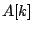):or, equivalently,whereis the fundamental frequency of the waveform, and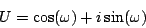is the unit-magnitude complex number whose argument is.

To analyze the first symmetry we delay the signalby a half-cycle. Sincewe get: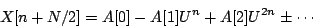In effect, a half-cycle delay changes the sign of every other term in the Fourier series. We combine this with the original series in two different ways. Letting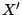denote half the sum of the two:andhalf the difference: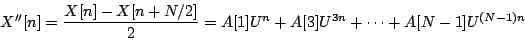we see thatconsists only of even-numbered harmonics (including DC) andonly of odd ones.

Furthermore, if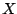happens to be equal to itself shifted a half cycle, that is, if, then (looking at the definitions ofand) we get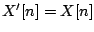and. This implies that, in this case,has only even numbered harmonics. Indeed, this should be no surprise, since in this casewould have to repeat every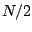samples, so its fundamental frequency is twice as high as normal for period.

In the same way, if], thencan have only odd-numbered harmonics. This allows us easily to split any desired waveform into its even- and odd-numbered harmonics. (This is equivalent to using a comb filter to extract even or odd harmonics; see Chapter 7.)

To derive the second symmetry relation we comparewith its time reversal,(or, equivalently, sincerepeats everysamples, with). The Fourier series becomes:(since the cosine function is even and the sine function is odd). In the same way as before we can extract the cosines by formingas half the sum:andas half the difference divided by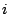: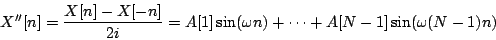So ifsatisfiesthe Fourier series consists of cosine terms only; ifit consists of sine terms only; and as before we can decompose any(that repeats everysamples) as a sum of the two.

SubsectionsNext: Sawtooth waves and symmetry Up: Classical waveforms Previous: Classical waveforms   Contents   Index
Miller Puckette 2006-12-30